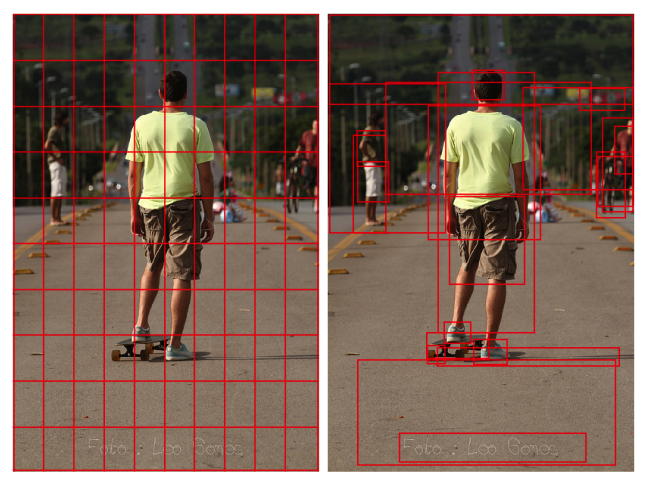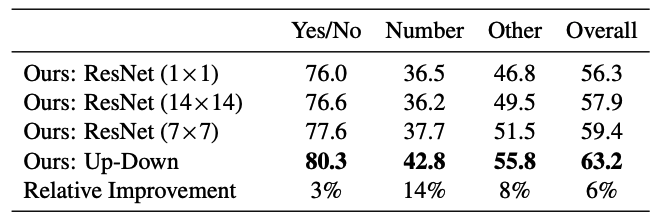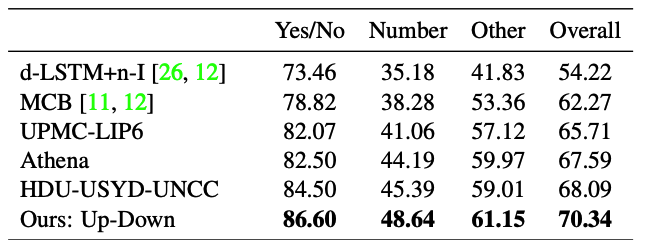# Bottom-Up and Top-Down Attention for Image Captioning and Visual Question Answering

## WHY?

In image captioning or visual question answering, the features of an image are extracted by the spatial output layer of pretrained CNN model.

## WHAT?

This paper suggests bottom-up attention using object detection model for extracting image features.Faster R-CNN in conjunction with ResNet101 is used followed by non-maximum supression using IOU threshold and the mean-pooling. The model was pretrained with ImageNet to classify object classes and trained additionally to predict the attribute classes.The VQA model of this paper is rather simple. This model utilizes the ‘gated tanh’ layer for non-linear transformation.

f_a(x) = \tilde{y}\circ g\\
\tilde{y} = tanh(Wx + b)\\
g = \sigma(W'x + b')\\

a_i = \mathbf{w}_a^{\top} f_a([\mathbf{v}_i, \mathbf{q}])\\
\mathbf{h} = f_q(\mathbf{q})\circ f_v(\hat{\mathbf{h}})\\
p(y) = \sigma(W_o f_o(\mathbf{h}))

## So?Bottm-up attention is shown to be useful than former methods.Up-Down model showed competitive results compared to other models in leader board of VQA 2.0 challenge (ensemble).

Powered by aiden